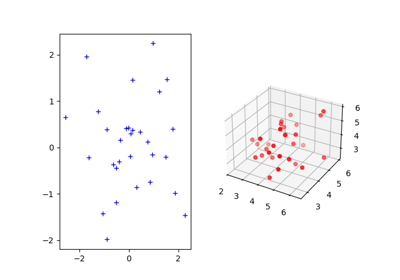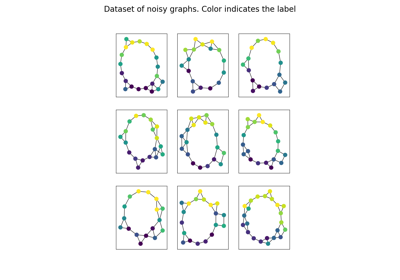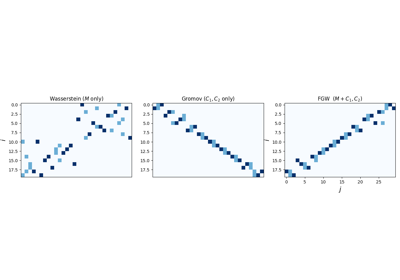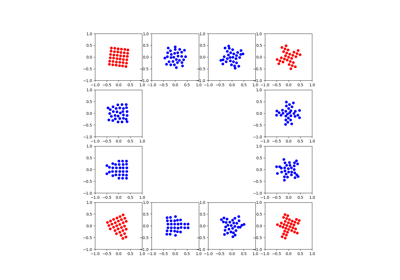# ot.gromov¶

Gromov-Wasserstein and Fused-Gromov-Wasserstein solvers

## Functions¶

ot.gromov.entropic_gromov_barycenters(N, Cs, ps, p, lambdas, loss_fun, epsilon, max_iter=1000, tol=1e-09, verbose=False, log=False, init_C=None)[source]

Returns the gromov-wasserstein barycenters of S measured similarity matrices

(Cs)_{s=1}^{s=S}

The function solves the following optimization problem:

$C = argmin_{C\in R^{NxN}} \sum_s \lambda_s GW(C,C_s,p,p_s)$

Where :

• $$C_s$$ : metric cost matrix

• $$p_s$$ : distribution

Parameters
• N (int) – Size of the targeted barycenter

• Cs (list of S np.ndarray of shape (ns,ns)) – Metric cost matrices

• ps (list of S np.ndarray of shape (ns,)) – Sample weights in the S spaces

• p (ndarray, shape(N,)) – Weights in the targeted barycenter

• lambdas (list of float) – List of the S spaces’ weights.

• loss_fun (callable) – Tensor-matrix multiplication function based on specific loss function.

• update (callable) – function(p,lambdas,T,Cs) that updates C according to a specific Kernel with the S Ts couplings calculated at each iteration

• epsilon (float) – Regularization term >0

• max_iter (int, optional) – Max number of iterations

• tol (float, optional) – Stop threshol on error (>0)

• verbose (bool, optional) – Print information along iterations.

• log (bool, optional) – Record log if True.

• init_C (bool | ndarray, shape (N, N)) – Random initial value for the C matrix provided by user.

Returns

C – Similarity matrix in the barycenter space (permutated arbitrarily)

Return type

ndarray, shape (N, N)

References

12

Peyré, Gabriel, Marco Cuturi, and Justin Solomon, “Gromov-Wasserstein averaging of kernel and distance matrices.” International Conference on Machine Learning (ICML). 2016.

ot.gromov.entropic_gromov_wasserstein(C1, C2, p, q, loss_fun, epsilon, max_iter=1000, tol=1e-09, verbose=False, log=False)[source]

Returns the gromov-wasserstein transport between (C1,p) and (C2,q)

(C1,p) and (C2,q)

The function solves the following optimization problem:

\begin{align}\begin{aligned}GW = arg\min_T \sum_{i,j,k,l} L(C1_{i,k},C2_{j,l})*T_{i,j}*T_{k,l}-\epsilon(H(T))\\s.t. T 1 = p\\ T^T 1= q\\ T\geq 0\end{aligned}\end{align}

Where : - C1 : Metric cost matrix in the source space - C2 : Metric cost matrix in the target space - p : distribution in the source space - q : distribution in the target space - L : loss function to account for the misfit between the similarity matrices - H : entropy

Parameters
• C1 (ndarray, shape (ns, ns)) – Metric cost matrix in the source space

• C2 (ndarray, shape (nt, nt)) – Metric costfr matrix in the target space

• p (ndarray, shape (ns,)) – Distribution in the source space

• q (ndarray, shape (nt,)) – Distribution in the target space

• loss_fun (string) – Loss function used for the solver either ‘square_loss’ or ‘kl_loss’

• epsilon (float) – Regularization term >0

• max_iter (int, optional) – Max number of iterations

• tol (float, optional) – Stop threshold on error (>0)

• verbose (bool, optional) – Print information along iterations

• log (bool, optional) – Record log if True.

Returns

T – Optimal coupling between the two spaces

Return type

ndarray, shape (ns, nt)

References

12

Peyré, Gabriel, Marco Cuturi, and Justin Solomon, “Gromov-Wasserstein averaging of kernel and distance matrices.” International Conference on Machine Learning (ICML). 2016.

### Examples using ot.gromov.entropic_gromov_wasserstein¶ot.gromov.entropic_gromov_wasserstein2(C1, C2, p, q, loss_fun, epsilon, max_iter=1000, tol=1e-09, verbose=False, log=False)[source]

Returns the entropic gromov-wasserstein discrepancy between the two measured similarity matrices

(C1,p) and (C2,q)

The function solves the following optimization problem:

$GW = \min_T \sum_{i,j,k,l} L(C1_{i,k},C2_{j,l})*T_{i,j}*T_{k,l}-\epsilon(H(T))$

Where : - C1 : Metric cost matrix in the source space - C2 : Metric cost matrix in the target space - p : distribution in the source space - q : distribution in the target space - L : loss function to account for the misfit between the similarity matrices - H : entropy

Parameters
• C1 (ndarray, shape (ns, ns)) – Metric cost matrix in the source space

• C2 (ndarray, shape (nt, nt)) – Metric costfr matrix in the target space

• p (ndarray, shape (ns,)) – Distribution in the source space

• q (ndarray, shape (nt,)) – Distribution in the target space

• loss_fun (str) – Loss function used for the solver either ‘square_loss’ or ‘kl_loss’

• epsilon (float) – Regularization term >0

• max_iter (int, optional) – Max number of iterations

• tol (float, optional) – Stop threshold on error (>0)

• verbose (bool, optional) – Print information along iterations

• log (bool, optional) – Record log if True.

Returns

gw_dist – Gromov-Wasserstein distance

Return type

float

References

12

Peyré, Gabriel, Marco Cuturi, and Justin Solomon, “Gromov-Wasserstein averaging of kernel and distance matrices.” International Conference on Machine Learning (ICML). 2016.

ot.gromov.fgw_barycenters(N, Ys, Cs, ps, lambdas, alpha, fixed_structure=False, fixed_features=False, p=None, loss_fun='square_loss', max_iter=100, tol=1e-09, verbose=False, log=False, init_C=None, init_X=None)[source]

Compute the fgw barycenter as presented eq (5) in .

Parameters
• N (integer) – Desired number of samples of the target barycenter

• Ys (list of ndarray, each element has shape (ns,d)) – Features of all samples

• Cs (list of ndarray, each element has shape (ns,ns)) – Structure matrices of all samples

• ps (list of ndarray, each element has shape (ns,)) – Masses of all samples.

• lambdas (list of float) – List of the S spaces’ weights

• alpha (float) – Alpha parameter for the fgw distance

• fixed_structure (bool) – Whether to fix the structure of the barycenter during the updates

• fixed_features (bool) – Whether to fix the feature of the barycenter during the updates

• loss_fun (str) – Loss function used for the solver either ‘square_loss’ or ‘kl_loss’

• max_iter (int, optional) – Max number of iterations

• tol (float, optional) – Stop threshol on error (>0).

• verbose (bool, optional) – Print information along iterations.

• log (bool, optional) – Record log if True.

• init_C (ndarray, shape (N,N), optional) – Initialization for the barycenters’ structure matrix. If not set a random init is used.

• init_X (ndarray, shape (N,d), optional) – Initialization for the barycenters’ features. If not set a random init is used.

Returns

• X (ndarray, shape (N, d)) – Barycenters’ features

• C (ndarray, shape (N, N)) – Barycenters’ structure matrix

• log_ (dict) – Only returned when log=True. It contains the keys: T : list of (N,ns) transport matrices Ms : all distance matrices between the feature of the barycenter and the other features dist(X,Ys) shape (N,ns)

References

24

Vayer Titouan, Chapel Laetitia, Flamary R{‘e}mi, Tavenard Romain and Courty Nicolas “Optimal Transport for structured data with application on graphs” International Conference on Machine Learning (ICML). 2019.

### Examples using ot.gromov.fgw_barycenters¶ot.gromov.fused_gromov_wasserstein(M, C1, C2, p, q, loss_fun='square_loss', alpha=0.5, armijo=False, log=False, **kwargs)[source]

Computes the FGW transport between two graphs see 

\begin{align}\begin{aligned}\begin{split}\gamma = arg\min_\gamma (1-\\alpha)*<\gamma,M>_F + \\alpha* \sum_{i,j,k,l} L(C1_{i,k},C2_{j,l})*T_{i,j}*T_{k,l}\end{split}\\s.t. \gamma 1 = p \gamma^T 1= q \gamma\geq 0\end{aligned}\end{align}

where : - M is the (ns,nt) metric cost matrix - p and q are source and target weights (sum to 1) - L is a loss function to account for the misfit between the similarity matrices

The algorithm used for solving the problem is conditional gradient as discussed in _

Parameters
• M (ndarray, shape (ns, nt)) – Metric cost matrix between features across domains

• C1 (ndarray, shape (ns, ns)) – Metric cost matrix representative of the structure in the source space

• C2 (ndarray, shape (nt, nt)) – Metric cost matrix representative of the structure in the target space

• p (ndarray, shape (ns,)) – Distribution in the source space

• q (ndarray, shape (nt,)) – Distribution in the target space

• loss_fun (str, optional) – Loss function used for the solver

• alpha (float, optional) – Trade-off parameter (0 < alpha < 1)

• armijo (bool, optional) – If True the steps of the line-search is found via an armijo research. Else closed form is used. If there is convergence issues use False.

• log (bool, optional) – record log if True

• **kwargs (dict) – parameters can be directly passed to the ot.optim.cg solver

Returns

• gamma (ndarray, shape (ns, nt)) – Optimal transportation matrix for the given parameters.

• log (dict) – Log dictionary return only if log==True in parameters.

References

24

Vayer Titouan, Chapel Laetitia, Flamary R{'e}mi, Tavenard Romain and Courty Nicolas “Optimal Transport for structured data with application on graphs”, International Conference on Machine Learning (ICML). 2019.

### Examples using ot.gromov.fused_gromov_wasserstein¶ot.gromov.fused_gromov_wasserstein2(M, C1, C2, p, q, loss_fun='square_loss', alpha=0.5, armijo=False, log=False, **kwargs)[source]

Computes the FGW distance between two graphs see 

\begin{align}\begin{aligned}\begin{split}\min_\gamma (1-\\alpha)*<\gamma,M>_F + \\alpha* \sum_{i,j,k,l} L(C1_{i,k},C2_{j,l})*T_{i,j}*T_{k,l}\end{split}\\s.t. \gamma 1 = p \gamma^T 1= q \gamma\geq 0\end{aligned}\end{align}

where : - M is the (ns,nt) metric cost matrix - p and q are source and target weights (sum to 1) - L is a loss function to account for the misfit between the similarity matrices The algorithm used for solving the problem is conditional gradient as discussed in _

Parameters
• M (ndarray, shape (ns, nt)) – Metric cost matrix between features across domains

• C1 (ndarray, shape (ns, ns)) – Metric cost matrix respresentative of the structure in the source space.

• C2 (ndarray, shape (nt, nt)) – Metric cost matrix espresentative of the structure in the target space.

• p (ndarray, shape (ns,)) – Distribution in the source space.

• q (ndarray, shape (nt,)) – Distribution in the target space.

• loss_fun (str, optional) – Loss function used for the solver.

• alpha (float, optional) – Trade-off parameter (0 < alpha < 1)

• armijo (bool, optional) – If True the steps of the line-search is found via an armijo research. Else closed form is used. If there is convergence issues use False.

• log (bool, optional) – Record log if True.

• **kwargs (dict) – Parameters can be directly pased to the ot.optim.cg solver.

Returns

• gamma (ndarray, shape (ns, nt)) – Optimal transportation matrix for the given parameters.

• log (dict) – Log dictionary return only if log==True in parameters.

References

24

Vayer Titouan, Chapel Laetitia, Flamary R{'e}mi, Tavenard Romain and Courty Nicolas “Optimal Transport for structured data with application on graphs” International Conference on Machine Learning (ICML). 2019.

ot.gromov.gromov_barycenters(N, Cs, ps, p, lambdas, loss_fun, max_iter=1000, tol=1e-09, verbose=False, log=False, init_C=None)[source]

Returns the gromov-wasserstein barycenters of S measured similarity matrices

(Cs)_{s=1}^{s=S}

The function solves the following optimization problem with block coordinate descent:

$C = argmin_C\in R^NxN \sum_s \lambda_s GW(C,Cs,p,ps)$

Where :

• Cs : metric cost matrix

• ps : distribution

Parameters
• N (int) – Size of the targeted barycenter

• Cs (list of S np.ndarray of shape (ns, ns)) – Metric cost matrices

• ps (list of S np.ndarray of shape (ns,)) – Sample weights in the S spaces

• p (ndarray, shape (N,)) – Weights in the targeted barycenter

• lambdas (list of float) – List of the S spaces’ weights

• loss_fun (tensor-matrix multiplication function based on specific loss function) –

• update (function(p,lambdas,T,Cs) that updates C according to a specific Kernel) – with the S Ts couplings calculated at each iteration

• max_iter (int, optional) – Max number of iterations

• tol (float, optional) – Stop threshol on error (>0).

• verbose (bool, optional) – Print information along iterations.

• log (bool, optional) – Record log if True.

• init_C (bool | ndarray, shape(N,N)) – Random initial value for the C matrix provided by user.

Returns

C – Similarity matrix in the barycenter space (permutated arbitrarily)

Return type

ndarray, shape (N, N)

References

12

Peyré, Gabriel, Marco Cuturi, and Justin Solomon, “Gromov-Wasserstein averaging of kernel and distance matrices.” International Conference on Machine Learning (ICML). 2016.

### Examples using ot.gromov.gromov_barycenters¶ot.gromov.gromov_wasserstein(C1, C2, p, q, loss_fun, log=False, armijo=False, **kwargs)[source]

Returns the gromov-wasserstein transport between (C1,p) and (C2,q)

The function solves the following optimization problem:

$GW = \min_T \sum_{i,j,k,l} L(C1_{i,k},C2_{j,l})*T_{i,j}*T_{k,l}$

Where : - C1 : Metric cost matrix in the source space - C2 : Metric cost matrix in the target space - p : distribution in the source space - q : distribution in the target space - L : loss function to account for the misfit between the similarity matrices

Parameters
• C1 (ndarray, shape (ns, ns)) – Metric cost matrix in the source space

• C2 (ndarray, shape (nt, nt)) – Metric costfr matrix in the target space

• p (ndarray, shape (ns,)) – Distribution in the source space

• q (ndarray, shape (nt,)) – Distribution in the target space

• loss_fun (str) – loss function used for the solver either ‘square_loss’ or ‘kl_loss’

• max_iter (int, optional) – Max number of iterations

• tol (float, optional) – Stop threshold on error (>0)

• verbose (bool, optional) – Print information along iterations

• log (bool, optional) – record log if True

• armijo (bool, optional) – If True the steps of the line-search is found via an armijo research. Else closed form is used. If there is convergence issues use False.

• **kwargs (dict) – parameters can be directly passed to the ot.optim.cg solver

Returns

• T (ndarray, shape (ns, nt)) –

Doupling between the two spaces that minimizes:

sum_{i,j,k,l} L(C1_{i,k},C2_{j,l})*T_{i,j}*T_{k,l}

• log (dict) – Convergence information and loss.

References

12

Peyré, Gabriel, Marco Cuturi, and Justin Solomon, “Gromov-Wasserstein averaging of kernel and distance matrices.” International Conference on Machine Learning (ICML). 2016.

13

Mémoli, Facundo. Gromov–Wasserstein distances and the metric approach to object matching. Foundations of computational mathematics 11.4 (2011): 417-487.

### Examples using ot.gromov.gromov_wasserstein¶ot.gromov.gromov_wasserstein2(C1, C2, p, q, loss_fun, log=False, armijo=False, **kwargs)[source]

Returns the gromov-wasserstein discrepancy between (C1,p) and (C2,q)

The function solves the following optimization problem:

$GW = \min_T \sum_{i,j,k,l} L(C1_{i,k},C2_{j,l})*T_{i,j}*T_{k,l}$

Where : - C1 : Metric cost matrix in the source space - C2 : Metric cost matrix in the target space - p : distribution in the source space - q : distribution in the target space - L : loss function to account for the misfit between the similarity matrices

Parameters
• C1 (ndarray, shape (ns, ns)) – Metric cost matrix in the source space

• C2 (ndarray, shape (nt, nt)) – Metric cost matrix in the target space

• p (ndarray, shape (ns,)) – Distribution in the source space.

• q (ndarray, shape (nt,)) – Distribution in the target space.

• loss_fun (str) – loss function used for the solver either ‘square_loss’ or ‘kl_loss’

• max_iter (int, optional) – Max number of iterations

• tol (float, optional) – Stop threshold on error (>0)

• verbose (bool, optional) – Print information along iterations

• log (bool, optional) – record log if True

• armijo (bool, optional) – If True the steps of the line-search is found via an armijo research. Else closed form is used. If there is convergence issues use False.

Returns

• gw_dist (float) – Gromov-Wasserstein distance

• log (dict) – convergence information and Coupling marix

References

12

Peyré, Gabriel, Marco Cuturi, and Justin Solomon, “Gromov-Wasserstein averaging of kernel and distance matrices.” International Conference on Machine Learning (ICML). 2016.

13

Mémoli, Facundo. Gromov–Wasserstein distances and the metric approach to object matching. Foundations of computational mathematics 11.4 (2011): 417-487.

ot.gromov.gwggrad(constC, hC1, hC2, T)[source]

The gradient is computed as described in Proposition 2 in .

Parameters
• constC (ndarray, shape (ns, nt)) – Constant C matrix in Eq. (6)

• hC1 (ndarray, shape (ns, ns)) – h1(C1) matrix in Eq. (6)

• hC2 (ndarray, shape (nt, nt)) – h2(C) matrix in Eq. (6)

• T (ndarray, shape (ns, nt)) – Current value of transport matrix T

Returns

Return type

ndarray, shape (ns, nt)

References

12

Peyré, Gabriel, Marco Cuturi, and Justin Solomon, “Gromov-Wasserstein averaging of kernel and distance matrices.” International Conference on Machine Learning (ICML). 2016.

ot.gromov.gwloss(constC, hC1, hC2, T)[source]

Return the Loss for Gromov-Wasserstein

The loss is computed as described in Proposition 1 Eq. (6) in .

Parameters
• constC (ndarray, shape (ns, nt)) – Constant C matrix in Eq. (6)

• hC1 (ndarray, shape (ns, ns)) – h1(C1) matrix in Eq. (6)

• hC2 (ndarray, shape (nt, nt)) – h2(C) matrix in Eq. (6)

• T (ndarray, shape (ns, nt)) – Current value of transport matrix T

Returns

loss – Gromov Wasserstein loss

Return type

float

References

12

Peyré, Gabriel, Marco Cuturi, and Justin Solomon, “Gromov-Wasserstein averaging of kernel and distance matrices.” International Conference on Machine Learning (ICML). 2016.

ot.gromov.init_matrix(C1, C2, p, q, loss_fun='square_loss')[source]

Return loss matrices and tensors for Gromov-Wasserstein fast computation

Returns the value of mathcal{L}(C1,C2) otimes T with the selected loss function as the loss function of Gromow-Wasserstein discrepancy.

The matrices are computed as described in Proposition 1 in 

Where :
• C1 : Metric cost matrix in the source space

• C2 : Metric cost matrix in the target space

• T : A coupling between those two spaces

The square-loss function L(a,b)=|a-b|^2 is read as :
L(a,b) = f1(a)+f2(b)-h1(a)*h2(b) with :
• f1(a)=(a^2)

• f2(b)=(b^2)

• h1(a)=a

• h2(b)=2*b

The kl-loss function L(a,b)=a*log(a/b)-a+b is read as :
L(a,b) = f1(a)+f2(b)-h1(a)*h2(b) with :
• f1(a)=a*log(a)-a

• f2(b)=b

• h1(a)=a

• h2(b)=log(b)

Parameters
• C1 (ndarray, shape (ns, ns)) – Metric cost matrix in the source space

• C2 (ndarray, shape (nt, nt)) – Metric costfr matrix in the target space

• T (ndarray, shape (ns, nt)) – Coupling between source and target spaces

• p (ndarray, shape (ns,)) –

Returns

• constC (ndarray, shape (ns, nt)) – Constant C matrix in Eq. (6)

• hC1 (ndarray, shape (ns, ns)) – h1(C1) matrix in Eq. (6)

• hC2 (ndarray, shape (nt, nt)) – h2(C) matrix in Eq. (6)

References

12

Peyré, Gabriel, Marco Cuturi, and Justin Solomon, “Gromov-Wasserstein averaging of kernel and distance matrices.” International Conference on Machine Learning (ICML). 2016.

ot.gromov.tensor_product(constC, hC1, hC2, T)[source]

Return the tensor for Gromov-Wasserstein fast computation

The tensor is computed as described in Proposition 1 Eq. (6) in .

Parameters
• constC (ndarray, shape (ns, nt)) – Constant C matrix in Eq. (6)

• hC1 (ndarray, shape (ns, ns)) – h1(C1) matrix in Eq. (6)

• hC2 (ndarray, shape (nt, nt)) – h2(C) matrix in Eq. (6)

Returns

tens – mathcal{L}(C1,C2) otimes T tensor-matrix multiplication result

Return type

ndarray, shape (ns, nt)

References

12

Peyré, Gabriel, Marco Cuturi, and Justin Solomon, “Gromov-Wasserstein averaging of kernel and distance matrices.” International Conference on Machine Learning (ICML). 2016.

ot.gromov.update_feature_matrix(lambdas, Ys, Ts, p)[source]

Updates the feature with respect to the S Ts couplings.

See “Solving the barycenter problem with Block Coordinate Descent (BCD)” in  calculated at each iteration

Parameters
• p (ndarray, shape (N,)) – masses in the targeted barycenter

• lambdas (list of float) – List of the S spaces’ weights

• Ts (list of S np.ndarray(ns,N)) – the S Ts couplings calculated at each iteration

• Ys (list of S ndarray, shape(d,ns)) – The features.

Returns

X

Return type

ndarray, shape (d, N)

References

24
Vayer Titouan, Chapel Laetitia, Flamary R{‘e}mi, Tavenard Romain

and Courty Nicolas

“Optimal Transport for structured data with application on graphs” International Conference on Machine Learning (ICML). 2019.

ot.gromov.update_kl_loss(p, lambdas, T, Cs)[source]

Updates C according to the KL Loss kernel with the S Ts couplings calculated at each iteration

Parameters
• p (ndarray, shape (N,)) – Weights in the targeted barycenter.

• lambdas (list of the S spaces' weights) –

• T (list of S np.ndarray of shape (ns,N)) – The S Ts couplings calculated at each iteration.

• Cs (list of S ndarray, shape(ns,ns)) – Metric cost matrices.

Returns

C – updated C matrix

Return type

ndarray, shape (ns,ns)

ot.gromov.update_square_loss(p, lambdas, T, Cs)[source]

Updates C according to the L2 Loss kernel with the S Ts couplings calculated at each iteration

Parameters
• p (ndarray, shape (N,)) – Masses in the targeted barycenter.

• lambdas (list of float) – List of the S spaces’ weights.

• T (list of S np.ndarray of shape (ns,N)) – The S Ts couplings calculated at each iteration.

• Cs (list of S ndarray, shape(ns,ns)) – Metric cost matrices.

Returns

C – Updated C matrix.

Return type

ndarray, shape (nt, nt)

ot.gromov.update_sructure_matrix(p, lambdas, T, Cs)[source]

Updates C according to the L2 Loss kernel with the S Ts couplings.

It is calculated at each iteration

Parameters
• p (ndarray, shape (N,)) – Masses in the targeted barycenter.

• lambdas (list of float) – List of the S spaces’ weights.

• T (list of S ndarray of shape (ns, N)) – The S Ts couplings calculated at each iteration.

• Cs (list of S ndarray, shape (ns, ns)) – Metric cost matrices.

Returns

C – Updated C matrix.

Return type

ndarray, shape (nt, nt)